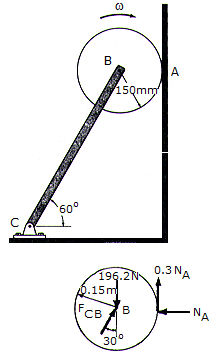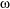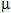# Engineering Mechanics - PKRB: Force and Animation - Discussion

### Discussion :: PKRB: Force and Animation - General Questions (Q.No.5)

5.The disk has a mass of 20 kg and is originally spinning at the end of the massless strut with an angular velocity of= 60 rad/s. If it is then placed against the wall, for whichA = 0.3, determine the time required for the motion to stop. What is the force in strut BC during this time?

 [A]. t = 3.11 s, FBC = 193.1 N [B]. t = 2.65 s, FBC = 227 N [C]. t = 5.30 s, FBC = 227 N [D]. t = 6.21 s, FBC = 193.1 N

Explanation:

No answer description available for this question.